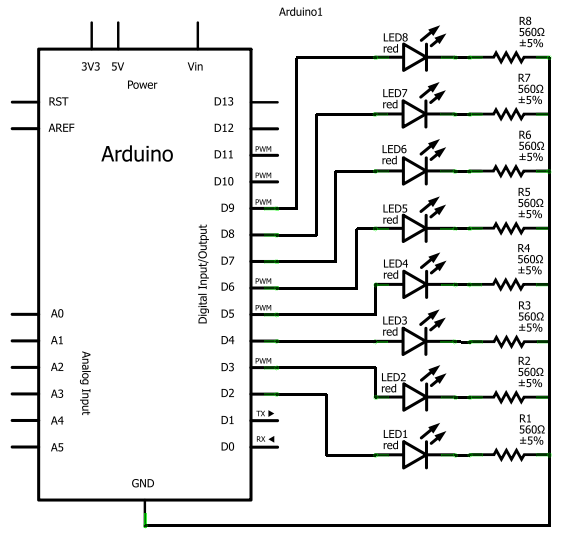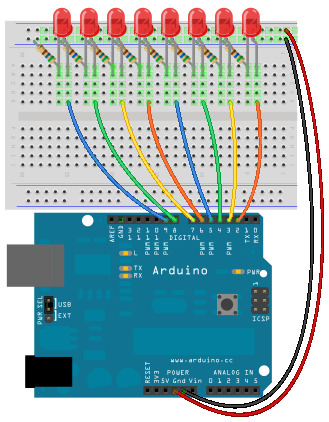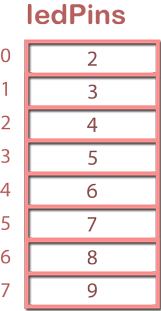# Lab 7: Arrays and LED's

## 1. Overview

In this lab, we do not look at any new hardware; the focus is entirely on software. We will implement a series of lights as an array and learn how to use `for` loops and functions with arrays.

## 2. First Build

It would be a really good idea to build this circuit before going to the lab because it is somewhat time consuming to connect all of the wires.

### 2.1 The Circuit

The circuit here is CIRC-02, from your book. For formality, the schematic when attached to the Arduino, looks like this:The breadboard will look like this:• The long end of the LED is connected via wire to the output pin
• Each LED has one hole between
• Each resistor has two holes between the next one (except where there are spaces between the groups of holes on the rails)

### 2.2 The Code

The full code for this circuit is available here: CIRC02-code.txt

You can use Command-a to select everything, Command-c to copy from your browser window, and Command-v to paste it into your Arduino window.

The code should look like this (although, some parts are missing). The focus of this excerpt is the code that runs immediately on the board:

```    ```
/*     ---------------------------------------------------------
*     |  Arduino Experimentation Kit Example Code             |
*     |  CIRC-02 .: 8 LED Fun :. (Multiple LEDs)              |
*     ---------------------------------------------------------
*
*  A few Simple LED animations
*
*
*/

//LED Pin Variables
int ledPins[] = {2,3,4,5,6,7,8,9}; //An array to hold the pin each LED is connected to
//i.e. LED #0 is connected to pin 2, LED #1, 3 and
//so on to address an array use ledPins this
//would equal 2 and ledPins would equal 9

/*
* setup() - this function runs once when you turn your Arduino on
* We the three control pins to outputs
*/
void setup()
{

//Set each pin connected to an LED to output mode (pulling high (on) or low (off)
for(int i = 0; i < 8; i++){   //this is a loop and will repeat eight times
pinMode(ledPins[i],OUTPUT);  //we use this to set each LED pin to output
}                               //It replaces 8 pin mode commands!

}

/*
* loop() - this function will start after setup finishes and then repeat
* we call a function called oneAfterAnother(). if you would like a different behaviour
* uncomment (delete the two slashes) one of the other lines
*/
void loop()                     // run over and over again
{
oneAfterAnotherNoLoop();   //this will turn on each LED one by one then turn each off

}

/*
* oneAfterAnotherNoLoop() - Will light one LED then delay for delayTime then light
* the next LED until all LEDs are on it will then turn them off one after another
*
* this does it without using a loop which makes for a lot of typing.
* oneOnAtATimeLoop() does exactly the same thing with less typing
*/
void oneAfterAnotherNoLoop(){
int delayTime = 100; //the time (in milliseconds) to pause between LEDs
//make smaller for quicker switching and larger for slower
digitalWrite(ledPins, HIGH);  //Turns on LED #0 (connected to pin 2 )
delay(delayTime);                //waits delayTime milliseconds
digitalWrite(ledPins, HIGH);  //Turns on LED #1 (connected to pin 3 )
delay(delayTime);                //waits delayTime milliseconds
digitalWrite(ledPins, HIGH);  //Turns on LED #2 (connected to pin 4 )
delay(delayTime);                //waits delayTime milliseconds
digitalWrite(ledPins, HIGH);  //Turns on LED #3 (connected to pin 5 )
delay(delayTime);                //waits delayTime milliseconds
digitalWrite(ledPins, HIGH);  //Turns on LED #4 (connected to pin 6 )
delay(delayTime);                //waits delayTime milliseconds
digitalWrite(ledPins, HIGH);  //Turns on LED #5 (connected to pin 7 )
delay(delayTime);                //waits delayTime milliseconds
digitalWrite(ledPins, HIGH);  //Turns on LED #6 (connected to pin 8 )
delay(delayTime);                //waits delayTime milliseconds
digitalWrite(ledPins, HIGH);  //Turns on LED #7 (connected to pin 9 )
delay(delayTime);                //waits delayTime milliseconds

//Turns Each LED Off
digitalWrite(ledPins, LOW);  //Turns on LED #0 (connected to pin 2 )
delay(delayTime);                //waits delayTime milliseconds
digitalWrite(ledPins, LOW);  //Turns on LED #1 (connected to pin 3 )
delay(delayTime);                //waits delayTime milliseconds
digitalWrite(ledPins, LOW);  //Turns on LED #2 (connected to pin 4 )
delay(delayTime);                //waits delayTime milliseconds
digitalWrite(ledPins, LOW);  //Turns on LED #3 (connected to pin 5 )
delay(delayTime);                //waits delayTime milliseconds
digitalWrite(ledPins, LOW);  //Turns on LED #4 (connected to pin 6 )
delay(delayTime);                //waits delayTime milliseconds
digitalWrite(ledPins, LOW);  //Turns on LED #5 (connected to pin 7 )
delay(delayTime);                //waits delayTime milliseconds
digitalWrite(ledPins, LOW);  //Turns on LED #6 (connected to pin 8 )
delay(delayTime);                //waits delayTime milliseconds
digitalWrite(ledPins, LOW);  //Turns on LED #7 (connected to pin 9 )
delay(delayTime);                //waits delayTime milliseconds
}
```
```

## 3. Hardware Theory

There is nothing new for hardware. You have seen it all before: LED's, resistors, and the Arduino. The code is the highlight of this lab! Get ready-the code is next.

## 4. Software Theory

### 4.1 One Dimensional Arrays

The following table compares an integer (from previous labs) to an array of integers.

Integer Array of Integers
Declaring and Initializing `int ledPin=13;` `int ledPins[] = {2,3,4,5,6,7,8,9};`
Pictorial RepresentationUsing `digitalWrite(ledPin, HIGH);`
``````
digitalWrite(ledPins, HIGH);
digitalWrite(ledPins, HIGH);
digitalWrite(ledPins, HIGH);
...
digitalWrite(ledPins, HIGH);```
```

#### Declaring and Initializing Arrays

Notice that in the declaration of an array:

`int ledPins[] = {2,3,4,5,6,7,8,9};`

1. We have square brackets ( `[]` ) after the `ledPins`. This is the way of indicating that we want to set aside contiguous (i.e. one after another) spaces of memory. We could also write the following to specify that we want to set aside eight spaces:
`int ledPins = {2,3,4,5,6,7,8,9};`

2. We are initializing the spaces in memory to the values 2, 3, 4, 5, 6, 7, 8, and 9. In our original statement (`int ledPins[]=...`), the compiler will know how many spaces are needed based on how many values we have.

#### Using Arrays

When we want to use `ledPins`, we have to remember that there are now eight spaces of memory (or eight values) associated with `ledPins`. We need a way of specifying which one of those eight spaces we want to access. This is where the indices (displayed on the left-hand side of the pictorial representation) are used. We put the index in square brackets after "ledPins".

For instance, if we want the value 8, we would write in the code:

`ledPins`

Some questions:

1. If we want the value 3, what would we write in the code?
2. What value will `ledPins` contain?
3. What value will `ledPins` contain?

### 4.2 For Loops with Arrays

Remember that `for` loops allow you to have a variable (maybe `i`) that starts at 0 and is incremented by 1 until it reaches a maximum (let us say 8). You should be thinking, "Wow, we can use for loops to control our index into the array". The `for` loop would look like this:

`for(int i = 0; i < 8; i++)`

You will notice that in the code that you copied into your Arduino window, there are a couple of places where `for` loops are used. The following is one usage (in `setup()`):

``````
for(int i = 0; i < 8; i++){         //this is a loop and will repeat eight times
pinMode(ledPins[i],OUTPUT);     //we use this to set each LED pin to output
} ``````

If you look inside the function: `oneAfterAnotherLoop()` you will find a couple of other similar usages of the `for` loop.

Try to predict what each of the following functions do:

1. `oneOnAtATime()`;
2. `inAndOut();`

Try running these functions by uncommenting them (i.e. delete the two slashes in front of them)!

To see the function running on its own, you might want to comment out all of the other function calls in `loop()`.

### 4.3 Passing Arrays to Functions

In the code that you are playing around with this week, you might want to specify a pattern for the LEDs. The pattern could be defined in an array containing values of `HIGH` and `LOW`. For instance, if you would like every second LED to be turned on, the "pattern" array would be:

`int pattern[] = {HIGH,LOW,HIGH,LOW,HIGH,LOW,HIGH,LOW};`

The code to turn the LEDs on or off in this pattern would be:

``````  for (int i=0; i<8; i++)
{
digitalWrite(ledPins[i], pattern[i]);
}
delay(3000);``````

Remember that `ledPins` were defined as:

`int ledPins[] = {2,3,4,5,6,7,8,9};`

Each cycle through the `for` loop will turn on (if `HIGH`) or off (if `LOW`) the LED starting at pin 2 and ending at pin 9 to yield the overall pattern at the end of the loop.

To take a look at the entire code for this example, click here.

So, you could have a couple of patterns and switch between one pattern and the next. If you have more than just a couple of patterns to switch between, your code will get longer than you might want it to be.

The next progression would be to send the pattern to a function. This will reduce the number of `for` loops that are necessary in your code to get the patterns to work. The following is some code which alternates between two patterns.

``````

//LED Pin Variables
int ledPins[] = {2,3,4,5}; //An array to hold the pin each LED is connected to

int pattern1[] = {HIGH,LOW,HIGH,LOW};
int pattern2[] = {LOW,HIGH,LOW,HIGH};

/* setup() - this function runs once when you turn your Arduino on
* We the three control pins to outputs
*/
void setup()
{

//Set each pin connected to an LED to output mode (pulling high (on) or low (off)
for(int i = 0; i < 4; i++)
{
pinMode(ledPins[i],OUTPUT);
}
}

/*
* loop() - this function will start after setup finishes and then repeat
*/
void loop()                     // run over and over again
{
makePattern(ledPins, pattern1, 4);
makePattern(ledPins, pattern2, 4);
}

/*
* makePattern - this function has three parameters:
*   leds[]-an array of output pins connected to LEDs
*   pattern[]-an array containing HIGH or LOW to indicate whether an LED is on or off
*   num-the number of LEDs
*/
void makePattern(int leds[], int pattern[], int num)
{
int delayTime = 200;
for(int i = 0; i < num; i++)
{
digitalWrite(leds[i], pattern[i]);
}
delay(delayTime);
}
```
```

Notice the function definition:

`void makePattern(int leds[], int pattern[], int num)`

Notice the call to the function:

`makePattern(ledPins, pattern1, 4);`

• the first argument is the array with the pin numbers that are connected to LEDs
• the second argument is the array containing the pattern of `HIGH` and `LOW`
• the third argument is how many LEDs you will be turning on or off

Pay attention to the difference between the function definition and the call to the function. The function definition needs to have what type of things you are using whereas the call to the function needs only the names of the arrays you want to use.

Some questions:

1. How many LEDs are being used in this code?
2. How would you get the other LEDs to have a pattern?

## 5. Exercise

Create a new function called `larsonScanner()`. A Larson Scanner looks like this: http://www.youtube.com/watch?v=NPAqGgYpb4A

The idea will be that it is sort of a mixture of the `oneAfterAnotherLoop()` and the `oneOnAtATime()` functions.

### Details:

Inside the new `larsonScanner` function:

• Use two `for` loops.
• Turn the lights on one at a time from index 0 to index 7, then turn the lights on one at a time from index 6 to index 1.
• Remember that you will have to turn off some lights too.

Create a new call in `loop()` to the `larsonScanner()` function.

### Deliverables:

All marking will be done in lab this week.
• Show the code for your Larson scanner.
• Demontrate the Larson scanner. There should be no hesitations or skips in the animation.
• Demonstrate your Challenge if you completed it.

### Challenge:

Modify the code for the `makePattern()` function, given in the notes above as:

`void makePattern(int leds[], int pattern[], int num)`

• Add a delay parameter to the function definition so that each time you call the function, you can send a different delay. Your new function might look like this:
`void makePattern(int leds[], int pattern[], int num, int delayT)`

• Define four patterns using eight LED pins. Note that the sample code only used four LED pins

• In the `loop()` function, call `makePattern` to display the four patterns that you have defined